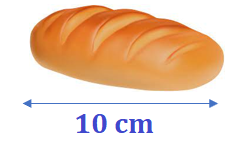# Math > Year 2

## Multiplication and division facts

Multiplication and division are related to each other. We can use multiplication facts to solve a division question and vice versa.

Nova cuts a loaf of bread of 10 cm into two equal parts. What will be the length of each part?We can use a multiplication fact to solve a division question.

10 shared into 2 groups is the same as 10÷ 2.

We know that 2 groups of 5 are 10.
5 × 2 = 10
So, each part is 5 cm.

Let's try one more example.

Harry has 15 chocolates. He decided to share it equally between 3 friends.

How many chocolates each one will have?

We can use multiplication facts to solve a division question.
15 shared into 3 groups is the same as 15 ÷ 3.
We know that 3 groups of 5 are 15.
5 × 3 = 15
So, each friend will have 5 chocolates.

Here is a question.

Edward says I can solve 70 ÷ 10 because I know my 7 times table really well.
Becca says I can solve 70 ÷ 10 because I know my 10 times table really well.

Who is correct?

1. Edward
2. Becca

3. None of them
4. Both of them

7 × 10 = 10 × 7 = 70

Using both of these multiplication facts we can solve 70 ÷ 10 = 7

So both of them are correct.

Hence, option 4 is the correct answer.

//